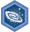## FANDOM

1,895 Pages

Algebra I: Expressions with rational exponents and radicalsDescription
Achieve mastery in all skills in Algebra I: Expressions with rational exponents and radicals
Details
TypeEnergy point(s) awarded 0
Status Available
Disclosed?
Repeating?

This page contains exercises which pertain to the subcategory Algebra I: Expressions with rational exponents and radicals. Mastering them awards the user with the Challenge Patch Algebra I: Expressions with rational exponents and radicals as well.

All items (18)

A
•Adding and subtracting radicals
E
•Extraneous solutions to radical equations
F
•Fractional exponents
•Fractional exponents 2
M
•Manipulating fractional exponents
•Multi-step simplification of rational exponent expressions
P
•Positive and negative exponents
•Properties of exponents
R
•Radical equations
•Rational exponents and radicals
S
•Simplifying expressions with exponents
•Simplifying rational expressions with exponent properties
•Simplifying square roots
•Simplifying square roots 2
•Single-step simplification of radical expressions
U
•Understanding fractional exponents
•Unit-fraction exponents and radicals
•Using exponent rules to evaluate expressions